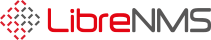# Change Calculation of Memory Utilization

Hi all,

We need to calculate the memory utilization like the below formula. How we can achieve this?

( 1 - ( ( {UCD-SNMP-MIB::memAvailReal.0} + {UCD-SNMP-MIB::memBuffer.0} + {UCD-SNMP-MIB::memCached.0} ) / {UCD-SNMP-MIB::memTotalReal.0} ) ) * 100

For example if the total real (physical) memory of one machine is 7393964, the available real memory is 1272980, the available buffer memory is 8456 and the available cached memory 646384 the Memory Utilization will be calculated like below.

(1 - ((1272980 + 8456 + 646384) / 7393964)) * 100 = 73.92 %